# Basics of k-NN Classification

### Import Necessary Modules

%matplotlib notebook
import numpy as np
import matplotlib
import matplotlib.pyplot as plt
import pandas as pd
import sklearn

print('       Numpy version ' + str(np.__version__))
print('  Matplotlib version ' + str(matplotlib.__version__))
print('      Pandas version ' + str(pd.__version__))
print('     Sklearn version ' + str(sklearn.__version__))

       Numpy version 1.17.4
Matplotlib version 3.1.1
Pandas version 0.25.3
Sklearn version 0.22.1


Open a small datafile with data related to fruit. Ity contains the mass, height, and width of a few samples of oranges, lemons, and apples. The heights were measured along the core, and the width was the largest dimension perpendicular to the height.

fruit_file = 'applied-classification/fruit_data_with_colors.txt'
sep='\t')
fruits.info()

<class 'pandas.core.frame.DataFrame'>
RangeIndex: 59 entries, 0 to 58
Data columns (total 7 columns):
fruit_label      59 non-null int64
fruit_name       59 non-null object
fruit_subtype    59 non-null object
mass             59 non-null int64
width            59 non-null float64
height           59 non-null float64
color_score      59 non-null float64
dtypes: float64(3), int64(2), object(2)
memory usage: 3.4+ KB

fruits.head()


fruit_label fruit_name fruit_subtype mass width height color_score
0 1 apple granny_smith 192 8.4 7.3 0.55
1 1 apple granny_smith 180 8.0 6.8 0.59
2 1 apple granny_smith 176 7.4 7.2 0.60
3 2 mandarin mandarin 86 6.2 4.7 0.80
4 2 mandarin mandarin 84 6.0 4.6 0.79

color_score maps from the numerical values to color categories as follows:

Color Category color_score
Red 0.85 - 1.00
Orange 0.75 - 0.85
Yellow 0.65 - 0.75
Green 0.45 - 0.65

The following mapping from label to name makes it easier to interpret results.

labels = fruits['fruit_label'].unique()
names = fruits['fruit_name'].unique()
lookup_fruit_name = dict(zip(labels, names))
lookup_fruit_name

{1: 'apple', 2: 'mandarin', 3: 'orange', 4: 'lemon'}


### Examine the Data

There are a few reaspons to examine the data as a first step:

1. Inspecting feature values will help identify what cleaning and preprocessing is still required.
2. The dataset may have missing data, noisy data, or inconsistencies such as the wrong data type being used for a column, incorrect units of measurement for a column, or too few examples of a particular class.
3. Inspecting the data may make it clear that theproblem is solvable without machine learning.

#### Feature Pair Plot

First, plot a feature pair plot. This shows all possible pairs of features and produces a scatter plot for each pair, showing how the featres are correlated to each other or not. It also has histograms of the features on the main diagonal.

This is an ideal visualization for a few reasons:

1. Immediately reveals the range of values as well as any outliers.
2. May give an idea as to how likely it is that a machine learning algorithm could do well at predicting the different classes. This is driven by how well clustered and separated the different types of objects are in feature space. “Feature space” refers to the represetnation of an object using specific features that are in certain columns of the data we have.

Not that a pair plot does not show interactions between all features that might exist, just between pairs of them.

from pandas.plotting import scatter_matrix


Matplotlib’s cm contains various colormaps, which are used to change the colors in plots.

from matplotlib import cm

cmap = cm.get_cmap('gnuplot')
scatter = scatter_matrix(fruits[['height',
'width',
'mass',
'color_score']],
c=fruits['fruit_label'],     # c is No longer listed as a parameter for panda's
marker='o',                  # scatter_matrix as of version 25.3, so likely to be
hist_kwds={'bins':15},       # deprecated soon.
figsize=(6,6),
cmap=cmap)

<IPython.core.display.Javascript object>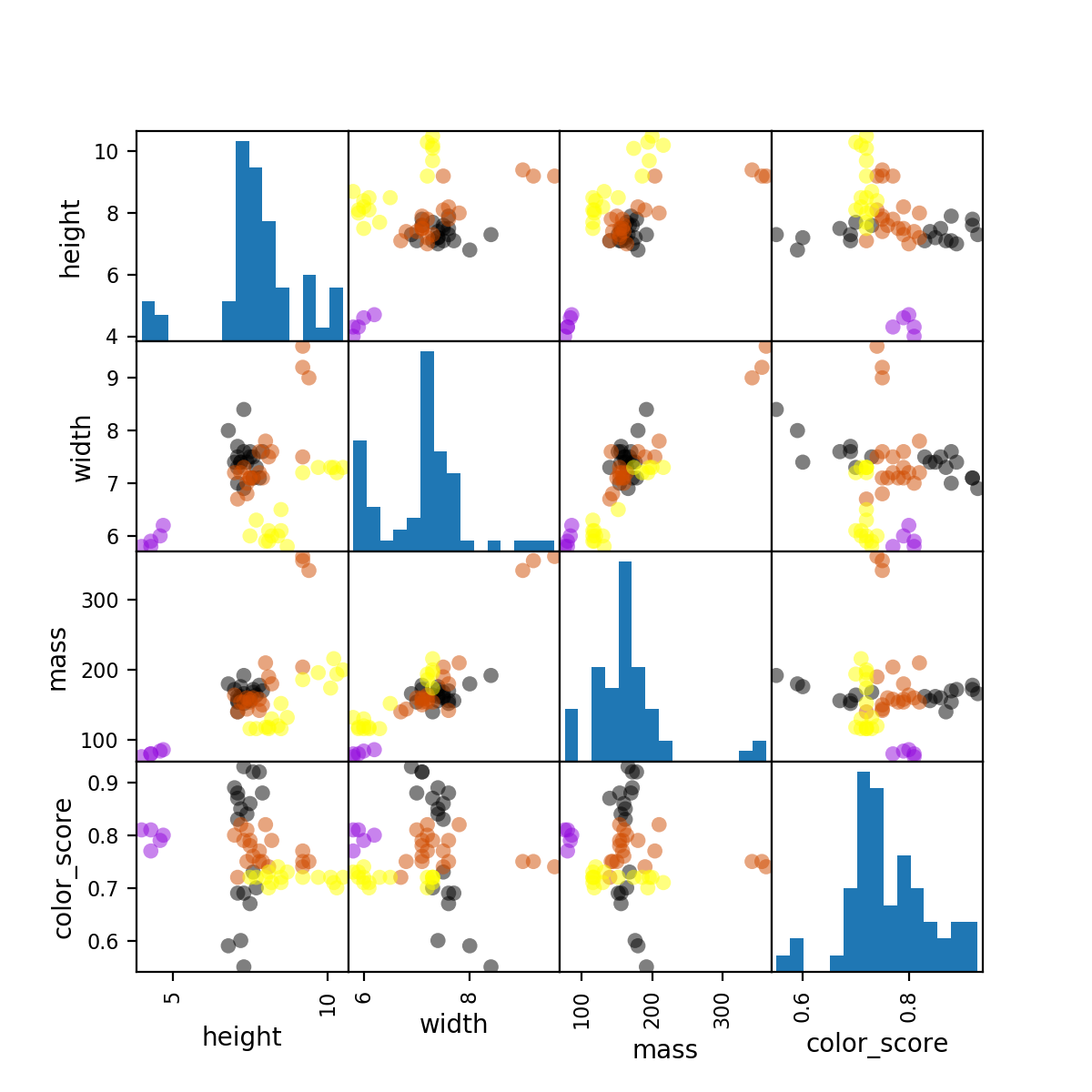#### 3D Scatterplot

We can also examine features that use a subset of three features by creating a three dimensional plot. We see below that the various fruits are well-separated in three-dimensional space as well.

from mpl_toolkits.mplot3d import Axes3D

fig = plt.figure()
projection = '3d')
ax.scatter(fruits['width'],
fruits['height'],
fruits['color_score'],
c = fruits['fruit_label'],
marker = 'o',
s=50)
ax.set_xlabel('width')
ax.set_ylabel('height')
ax.set_zlabel('color_score')
plt.show()

<IPython.core.display.Javascript object>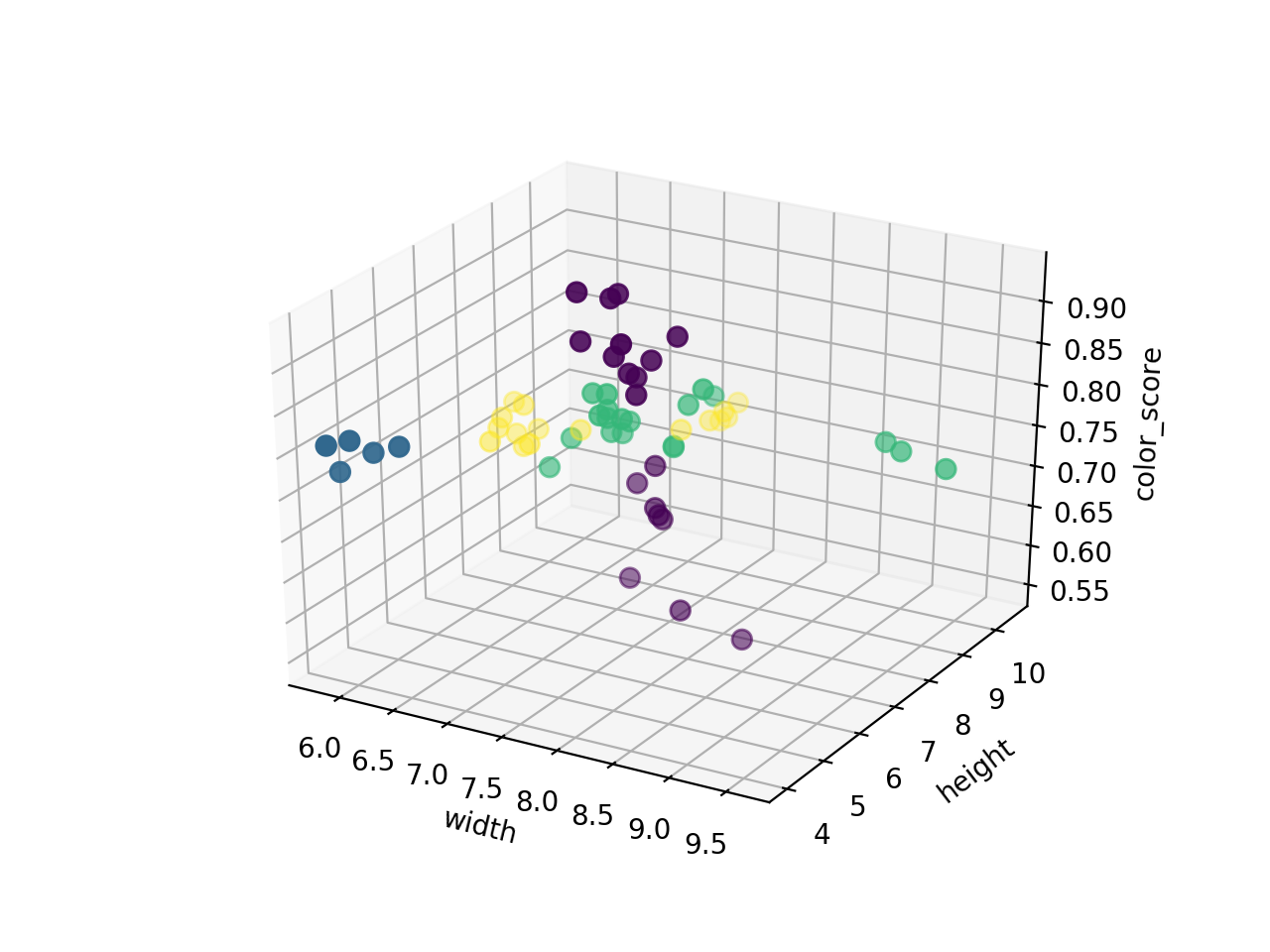### Create a Train-Test split

Scikit-Learn’s train_test_split is a means of splitting a dataset into training and testing sets. The training set is not allowed to be used to test the performance of the classifier, and vice versa.

from sklearn.model_selection import train_test_split


The naming convention shown below is typical:

• X: contains all columns of the dataset except the label.
• Y: contains the label.
• X_train, Y_train: contain data from the rows of the dataset that will be used to train the model.
• X_test, Y_test: contain data from the rows of the dataset that will be used to test the model.
X = fruits[['mass','width','height']]   # Input Data - excludes color
y = fruits['fruit_label']               # Data Labels
X_train, X_test, y_train, y_test = train_test_split(X,
y,
random_state=0)


The default is a 75% / 25% train-test split.

print('Train set size = ' + str(X_train.shape))
print(' Test set size = ' + str(X_test.shape))

Train set size = 44
Test set size = 15


### Create Classifier Object

Sklearn’s KNeighborsClassifer is a nearest neighbors classifier.

Nearest Neighbors classifers can be used for both classification and regression. They are an example of “instance based” or “memory based” supervised learning. This means that these methods memorize the labeled examples that they see in the training set and use those memorized examples to classify new objects later.

The “k” in “k-NN” stands for the number of examples that have the closest features. When a trained k-NN classifier receives a new object to make a prediction on, it looks at the nearest k objects to determine how to classify the new object. So, if k is 1, it looks at the nearest single object. If k is greater than 1, then it looks at the k nearest neighbors and the label is a simple majority vote. For this reason, k is typically an odd number.

A nearest neighbor algorithm needs four things specified:

1. A distance metric (most commonly, Euclidean),
2. How many “nearest” neighbors to use (for example, 5),
3. Optional weighting functions on the neighbor points (ignored), and
4. Method for aggregating the classes of neighbor points (simple majority vote).
from sklearn.neighbors import KNeighborsClassifier

knn = KNeighborsClassifier(n_neighbors = 5)


Train the classifier using the training data.

The k-NN classifier is a specific example of a more general class in Scikit-Learn called “Estimators.” All estimators have a fit method that is used to train an estimator on a set of training data.

knn.fit(X_train, y_train)

KNeighborsClassifier(algorithm='auto', leaf_size=30, metric='minkowski',
metric_params=None, n_jobs=None, n_neighbors=5, p=2,
weights='uniform')


Estimate the accuracy of the classifier on future data using the test data.

knn.score(X_test, y_test)

0.5333333333333333


Use the trained classifier model to classify new, previously unseen objects.

For example: a small fruit with mass 20g, width 4.3 cm, height 5.5 cm.

prediction = knn.predict([[20, 4.3, 5.5]])
lookup_fruit_name[prediction]

'mandarin'


Another example: a larger, elongated fruit with mass 100g, width 6.3 cm, height 8.5 cm.

prediction = knn.predict([[100, 6.3, 8.5]])
lookup_fruit_name[prediction]

'lemon'


### How sensitive is the k-NN classification accuracy to the choice of the “K” parameter?

In general, the best value for k is the value that leans to the best accuracy of the classifier. This can vary significantly depending on the dataset. This dataset performs worse with larger values of k, but the best approach to optimizing k would be to vary k while also varying the size of the train-test splits.

k_range = range(1,25)
scores = []

for k in k_range:
knn = KNeighborsClassifier(n_neighbors = k)
knn.fit(X_train, y_train)
scores.append(knn.score(X_test, y_test))

plt.figure()
plt.xlabel('k')
plt.ylabel('accuracy')
plt.scatter(k_range, scores)
plt.xticks([0,5,10,15,20,25])
plt.ylim(0,1);

<IPython.core.display.Javascript object>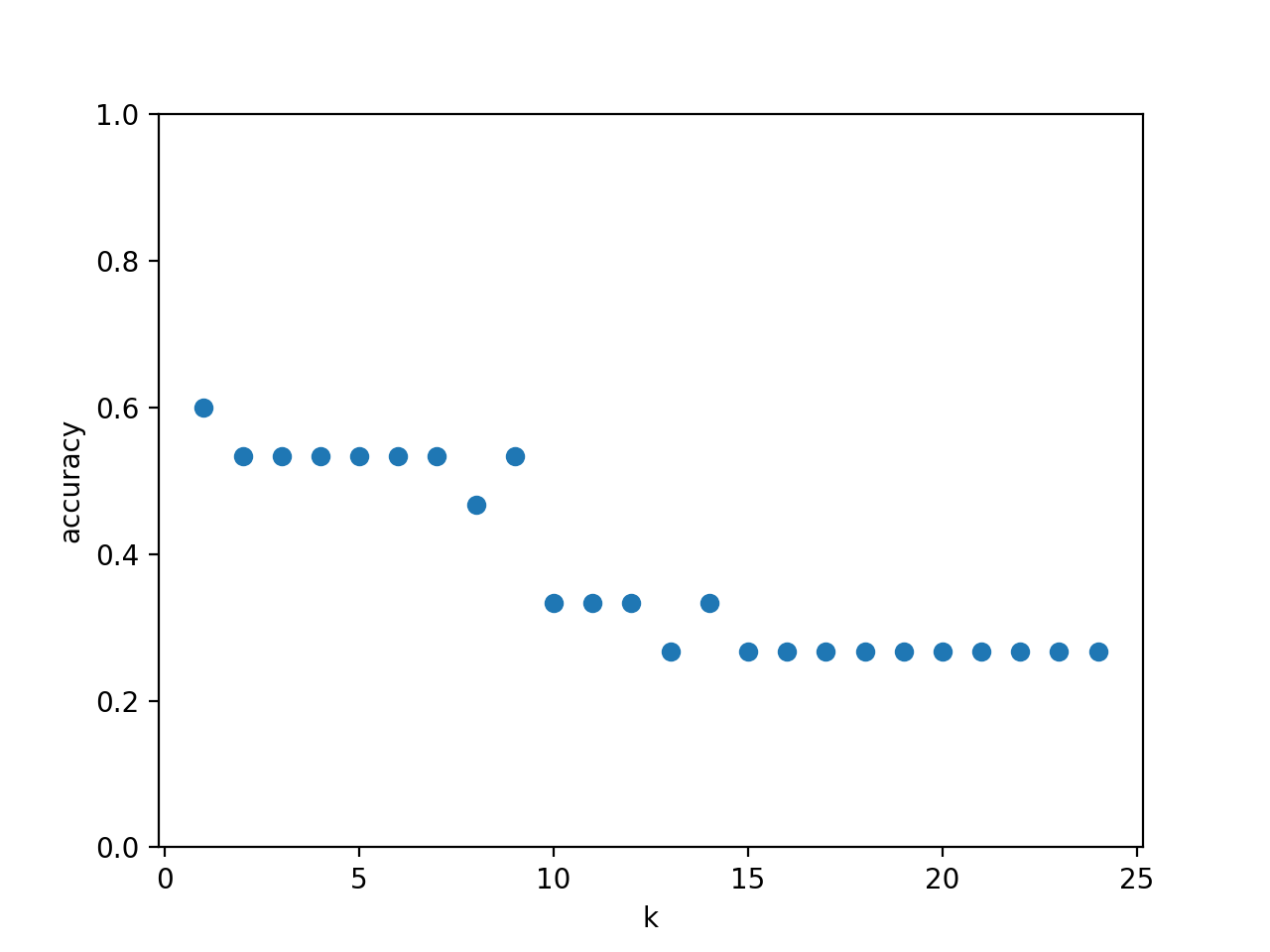### How sensitive is the k-NN classification accuracy to the train-test split proportion?

t = np.arange(0.1,0.95,0.05)

knn = KNeighborsClassifier(n_neighbors = 5)

plt.figure()

for s in t:
scores = []
for i in range(1,1000):
X_train, X_test, y_train, y_test = train_test_split(X,
y,
test_size=1-s)
knn.fit(X_train, y_train)
scores.append(knn.score(X_test, y_test))
plt.plot(s,
np.mean(scores),
'bo')

plt.xlabel('Training set proportion (%)')
plt.ylabel('Accuracy')
plt.ylim(0,1)
plt.xlim(0,1);

<IPython.core.display.Javascript object>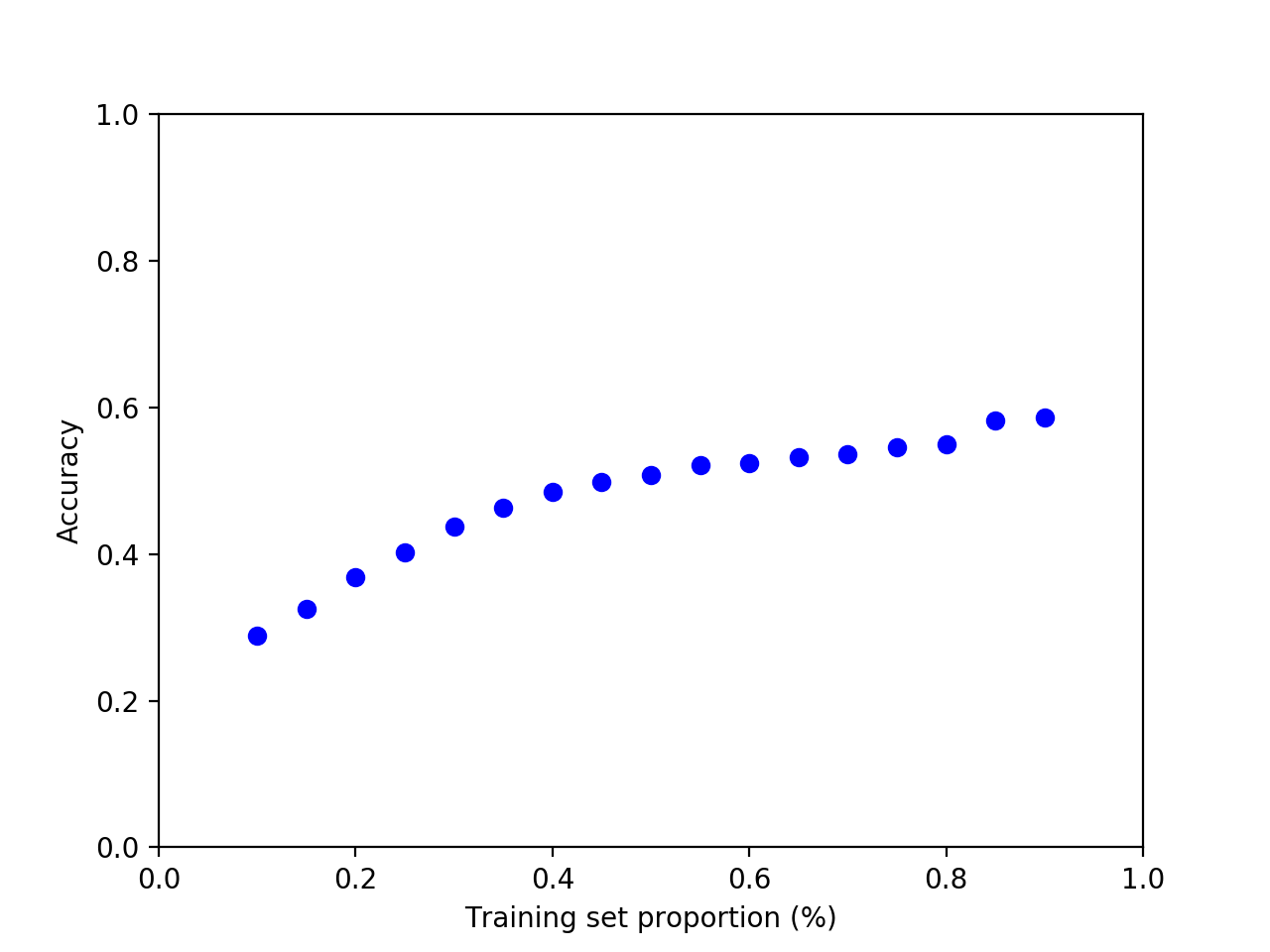### Plot the Decision Boundaries

from matplotlib.colors import ListedColormap
import matplotlib.patches as mpatches

def plot_fruit_knn(X, y, n_neighbors, weights):
X_mat = X[['height', 'width']].values
y_mat = y.values

# Create color maps
cmap_light = ListedColormap(['#FFAAAA', '#AAFFAA', '#AAAAFF','#AFAFAF'])
cmap_bold  = ListedColormap(['#FF0000', '#00FF00', '#0000FF','#AFAFAF'])

clf = KNeighborsClassifier(n_neighbors,
weights=weights)
clf.fit(X_mat, y_mat)

# Plot the decision boundary by assigning a color in the color map
# to each mesh point.

mesh_step_size = .01  # step size in the mesh
plot_symbol_size = 50

x_min, x_max = X_mat[:, 0].min() - 1, X_mat[:, 0].max() + 1
y_min, y_max = X_mat[:, 1].min() - 1, X_mat[:, 1].max() + 1
xx, yy = np.meshgrid(np.arange(x_min, x_max, mesh_step_size),
np.arange(y_min, y_max, mesh_step_size))
Z = clf.predict(np.c_[xx.ravel(), yy.ravel()])

# Put the result into a color plot
Z = Z.reshape(xx.shape)
plt.figure()
plt.pcolormesh(xx,
yy,
Z,
cmap=cmap_light)

# Plot training points
plt.scatter(X_mat[:, 0],
X_mat[:, 1],
s=plot_symbol_size,
c=y,
cmap=cmap_bold,
edgecolor = 'black')
plt.xlim(xx.min(), xx.max())
plt.ylim(yy.min(), yy.max())
plt.title("k = {}, weights = {}".format(n_neighbors,
weights))

patch0 = mpatches.Patch(color='#FF0000', label='apple')
patch1 = mpatches.Patch(color='#00FF00', label='mandarin')
patch2 = mpatches.Patch(color='#0000FF', label='orange')
patch3 = mpatches.Patch(color='#AFAFAF', label='lemon')
plt.legend(handles=[patch0, patch1, patch2, patch3])

plt.xlabel('height (cm)')
plt.ylabel('width (cm)')

plt.show()


The “uniform” parameter passed to the function above means to treat all neighbors uniformly. Another option would be to pass in the word “distance” which would weight the neighbors by their distance from the predict point.

For small values of k, like 1, the prediction is sensitive to noise, outliers, mislabeled data and other sources of variation. For larger values of k the areas are smoother and not as fragmented and more robust to noise in the individual points, but possibly with some mistakes.

This is known as a bias/variance tradeoff.

X_train, X_test, y_train, y_test = train_test_split(X,
y,
random_state=0)
for k, w in [(1,'uniform'),
(3,'uniform'),
(3,'distance'),
(5,'uniform'),
(5,'distance'),
(7,'uniform'),
(7,'distance')]:
plot_fruit_knn(X_train,
y_train,
k,
w)

<IPython.core.display.Javascript object>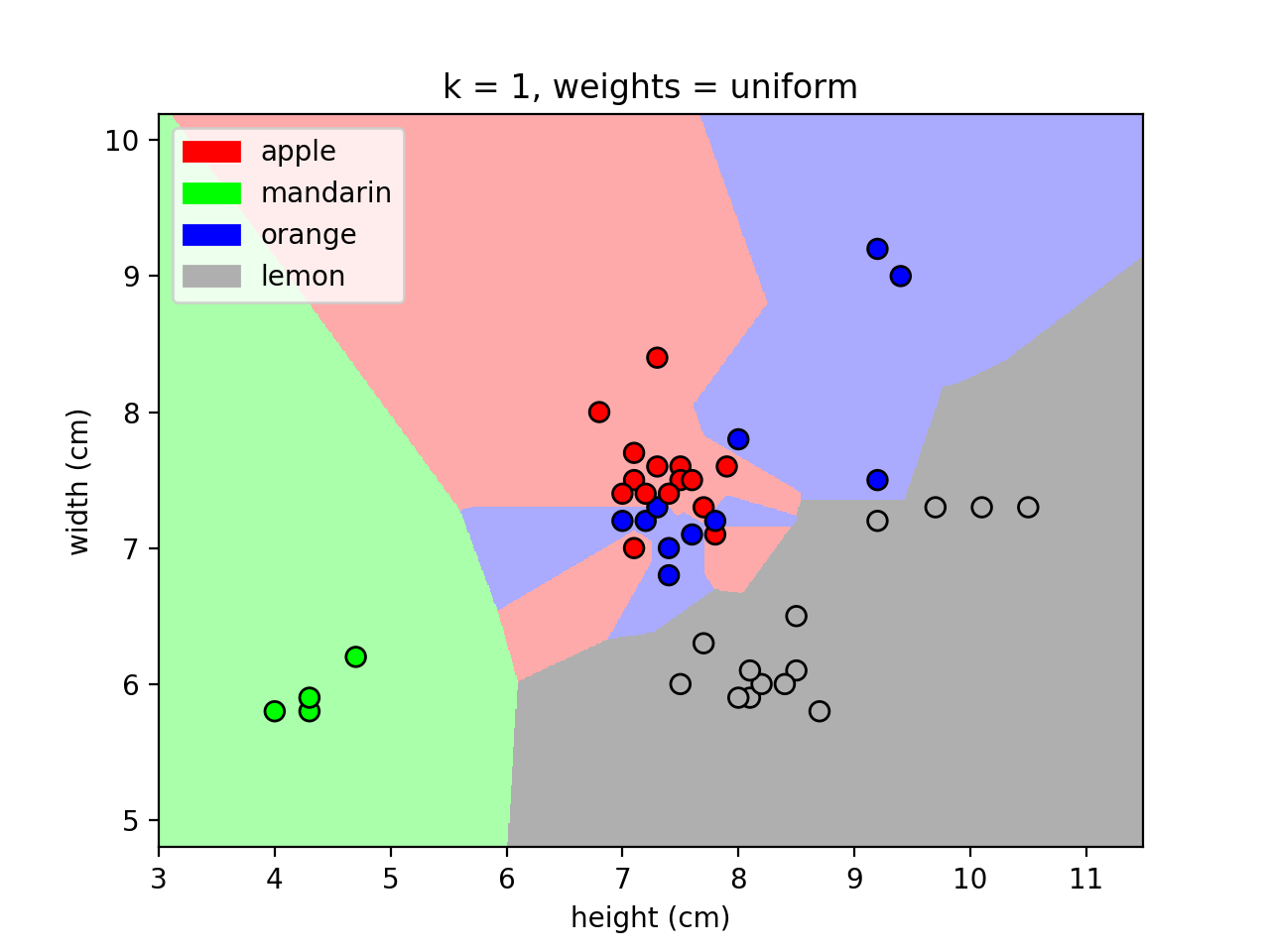<IPython.core.display.Javascript object>